# Shear Strength

The following strength criteria are available in CPillar for defining the strength of your rock mass:

• Mohr-Coulomb
• Hoek-Brown
• Generalized Hoek-Brown

## Mohr-Coulomb

For the Mohr-Coulomb criterion you must define the following parameters for each analysis type:

• Rigid analysis: Cohesion, Friction Angle
• Elastic analysis: Cohesion, Friction Angle, Rock Mass Modulus
• Voussoir analysis: Intact Strength, Rock Mass Cohesion, Rock Mass Friction Angle, Rock Mass Modulus, Poisson Ratio

NOTE: Intact Strength is not required when using the Mohr-Coulomb strength criterion with a Rigid or Elastic analysis. For a Voussoir analysis, the Intact Strength is required to calculate the safety factor for localized crushing failure, and therefore it is a required parameter regardless of Strength Criterion.

## Hoek-Brown

The Hoek-Brown criterion in CPillar refers to the original Hoek-Brown failure criterion [Hoek & Bray (1981)], described by the following equation: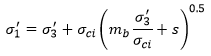Note that this is a special case of the Generalized Hoek-Brown criterion, with the constant a = 0.5. See below for the definition of the parameters in this equation.

The original Hoek-Brown criterion has been found to work well for most rocks of good to reasonable quality in which the rock mass strength is controlled by tightly interlocking angular rock pieces.

For lesser quality rock masses, the Generalized Hoek-Brown criterion can be used.

For the Hoek-Brown criterion, you must define the following parameters for each analysis type:

• Rigid analysis: Intact Strength, Rock Mass m Value, Rock Mass s Value
• Elastic analysis: Intact Strength, Rock Mass m Value, Rock Mass s Value, Rock Mass Modulus
• Voussoir analysis: Intact Strength, Rock Mass m Value, Rock Mass s Value, Rock Mass Modulus, Poisson Ratio

## Generalized Hoek-Brown (mb, s, a)

For the Generalized Hoek-Brown (mb, s, a) criterion you must define the following parameters for each analysis type:

• Rigid analysis: Intact Strength, Rock Mass mb Value, Rock Mass s Value, Rock Mass a Value
• Elastic analysis: Intact Strength, Rock Mass mb Value, Rock Mass s Value, Rock Mass a Value, Rock Mass Modulus
• Voussoir analysis: Intact Strength, Rock Mass mb Value, Rock Mass s Value, Rock Mass a Value, Rock Mass Modulus, Poisson Ratio

The Generalized Hoek-Brown strength criterion is described by the following equation: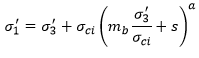where:

• mb is a reduced value (for the rock mass) of the material constant mi (for the intact rock)
• s and a are constants which depend upon the characteristics of the rock mass
•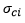is the uniaxial compressive strength (UCS) of the intact rock pieces
•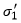andare the axial and confining effective principal stresses respectively

## Generalized Hoek-Brown (GSI, mi, D)

In most cases it is practically impossible to carry out triaxial or shear tests on rock masses at a scale which is necessary to obtain direct values of the parameters in the Generalized Hoek-Brown equation. Therefore some practical means of estimating the material constants mb, s, and a is required. According to the latest research, the parameters of the Generalized Hoek-Brown criterion (Hoek, Carranza-Torres, & Corkum, 2002), can be determined from the following equations: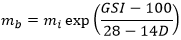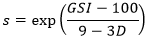where:

• GSI is the Geological Strength Index
• mi is a material constant for the intact rock
• the parameter D is a "disturbance factor" which depends upon the degree of disturbance to which the rock mass has been subjected by blast damage and stress relaxation. It varies from 0 for undisturbed in situ rock masses to 1 for very disturbed rock masses.